# java泛型

java泛型(generics)是jdk5引入的新特性,泛型提供了编译时类型安全检测机制,该机制运行开发者在编译时检测到非法的类型.

``````public class TestGeneric<T> {
private T t;
public void setT(T t) {this.t = t;}
public T getT() {return t;}
public static void main(String[] args) {
//这里直接指定String作为参数类型
TestGeneric<String> testGeneric = new TestGeneric<>();
//setT时,T就是String
testGeneric.setT("test");
//可以直接打印getT(),默认为String
System.out.println(testGeneric.getT());
}
}
``````

# 泛型中的通配符

• K和V 表示key,value,比如用于map的通配符
• T(type) 表示具体的一个java类型
• E (element) 表示一个元素,例如List

## 上界通配符 `<T extends E>`

``````    public static void main(String[] args) {
//这里直接指定String作为参数类型
TestGeneric<String> testGeneric = new TestGeneric<>();
HashMap<String, Integer> hashMap = new HashMap<>();
hashMap.put("a",1);
Map map = testGeneric.test(hashMap);
System.out.println(map.get("a"));
}
public <E extends Map> E test( E e) {
return e;
}
``````

## 下界通配符`<? super E>`

``````private <T> void test(List<? super T> dst, List<T> src){
for (T t : src) {
}
}

public static void main(String[] args) {
List<Dog> dogs = new ArrayList<>();
List<Animal> animals = new ArrayList<>();
new Test3().test(animals,dogs);
}
// Dog 是 Animal 的子类
class Dog extends Animal {

}
``````

## 特殊的?通配符

?和T都表示不确定的类型,区别在于一个是字母,可以直接操作,但是对? 不能直接操作,比如:

``````// 可以
T t = operate();

// 不可以
？car = operate();
``````

##### 热门推荐
•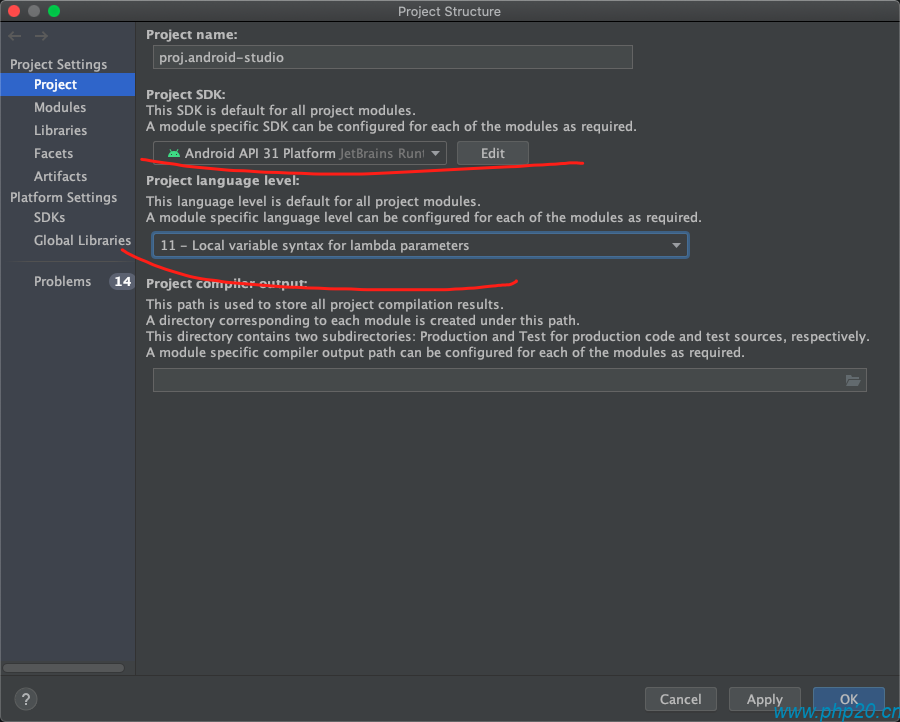浏览(1,986)
•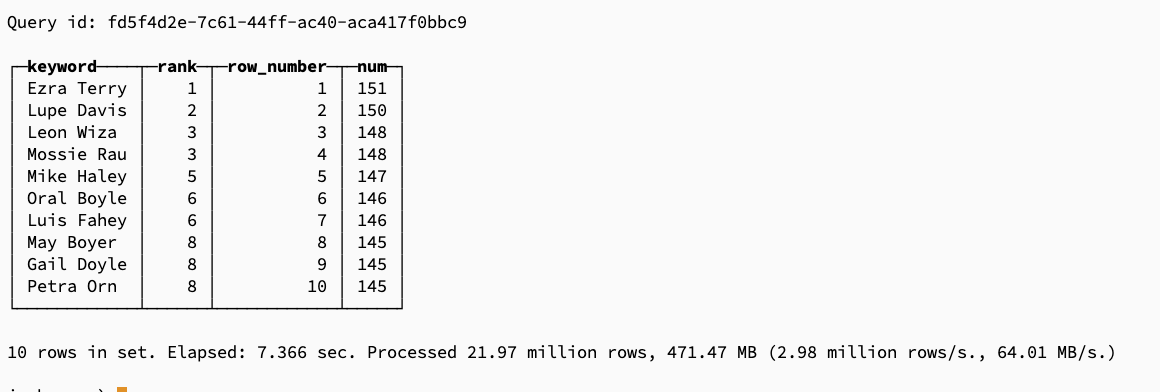浏览(791)
•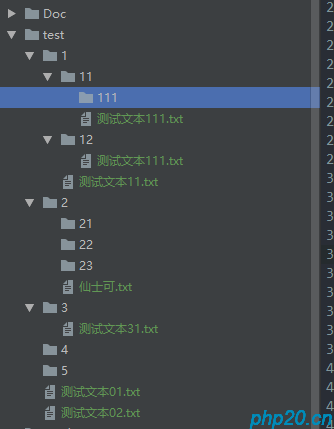浏览(782)
••浏览(685)
•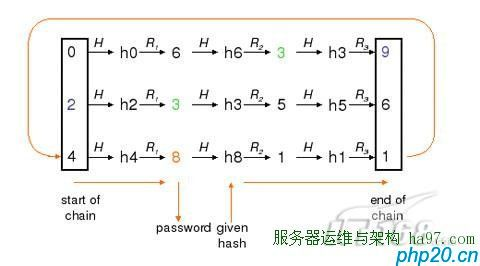•浏览(641)
•浏览(539)
•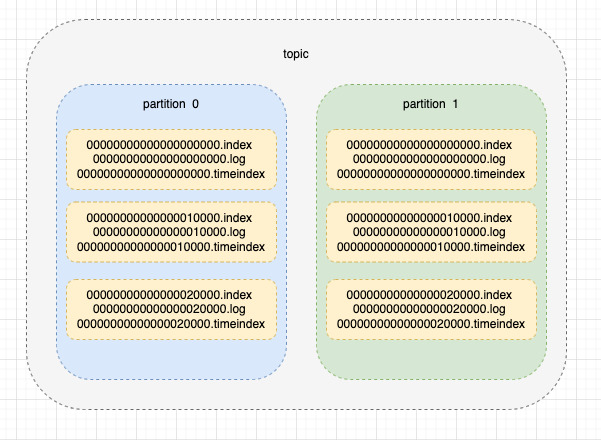浏览(487)
•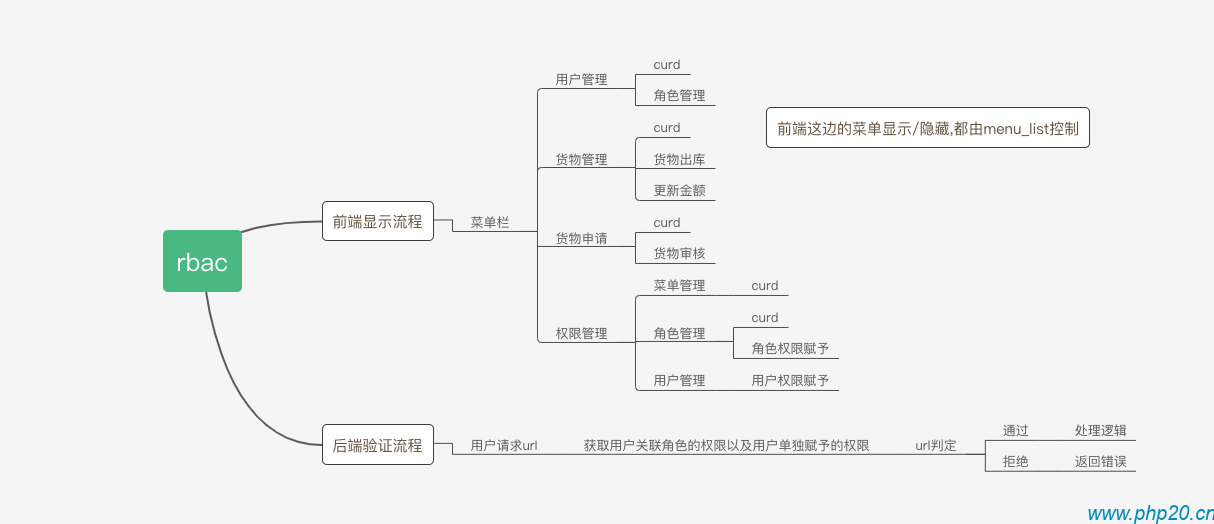浏览(477)